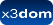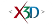## Node: SolidOfRevolution

 X3DOM Component: Geometry3DExt

➨ Fields
Describes a SolidOfRevolution shape.

### HTML Encoding and Default Values⤴

``` <SolidOfRevolution angle='2*Math.PI' caps='true' ccw='true' creaseAngle='0' crossSection='[]' lit='true' metadata='X3DMetadataObject' solid='true' subdivision='32' useGeoCache='true' ></SolidOfRevolution> ```

### Fields⤴

These are the X3D / X3DOM fields of this node. Values should usually be received / set as strings via DOM functions (i.e., using `setAttribute("myFieldName", "myFieldValue")` and `getAttribute("myFieldName")`).
Name Type Default Value Range Inheritance Standard Description
angle SFFloat PI [0, inf]The subtended angle through which the 2D loop is swept around the x axis.
caps SFBool trueSpecifies whether the caps exist.
ccw SFBool true Rendering/X3DGeometryNodeThe ccw field defines the ordering of the vertex coordinates of the geometry with respect to user-given or automatically generated normal vectors used in the lighting model equations.
creaseAngle SFFloat 0 [0, inf]The creaseAngle field affects how default normals are generated. If the angle between the geometric normals of two adjacent faces is less than the crease angle, normals shall be calculated so that the faces are shaded smoothly across the edge; otherwise, normals shall be calculated so that a lighting discontinuity across the edge is produced. Crease angles shall be greater than or equal to 0.0 angle base units.
crossSection MFVec2f []Defines the cross section that is swept around the axis. The cross section is described as an array of 2D vertices.
lit SFBool true Rendering/X3DGeometryNodeSpecifies whether this geometry should be rendered with or without lighting.
metadata SFNode X3DMetadataObject Core/X3DNodeField to add metadata information
solid SFBool true Rendering/X3DGeometryNodeSpecifies whether backface-culling is used. If solid is TRUE only front-faces are drawn.
subdivision SFFloat 32Specifies the number of steps that are generated to approximate the shape.
useGeoCache SFBool true Rendering/X3DGeometryNodeMost geo primitives use geo cache and others might later on, but one should be able to disable cache per geometry node. Defaults to global useGeoCache setting parameter.